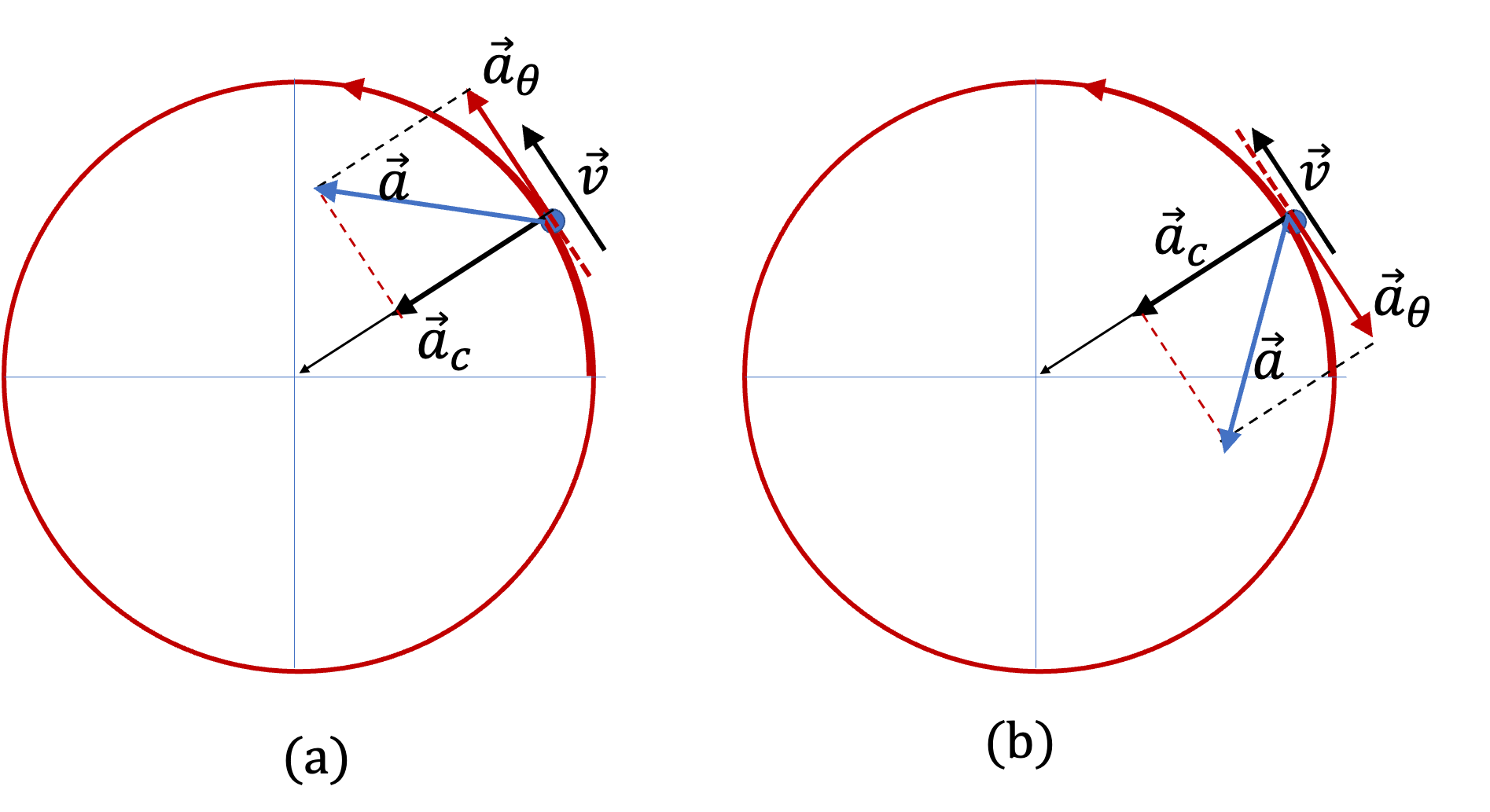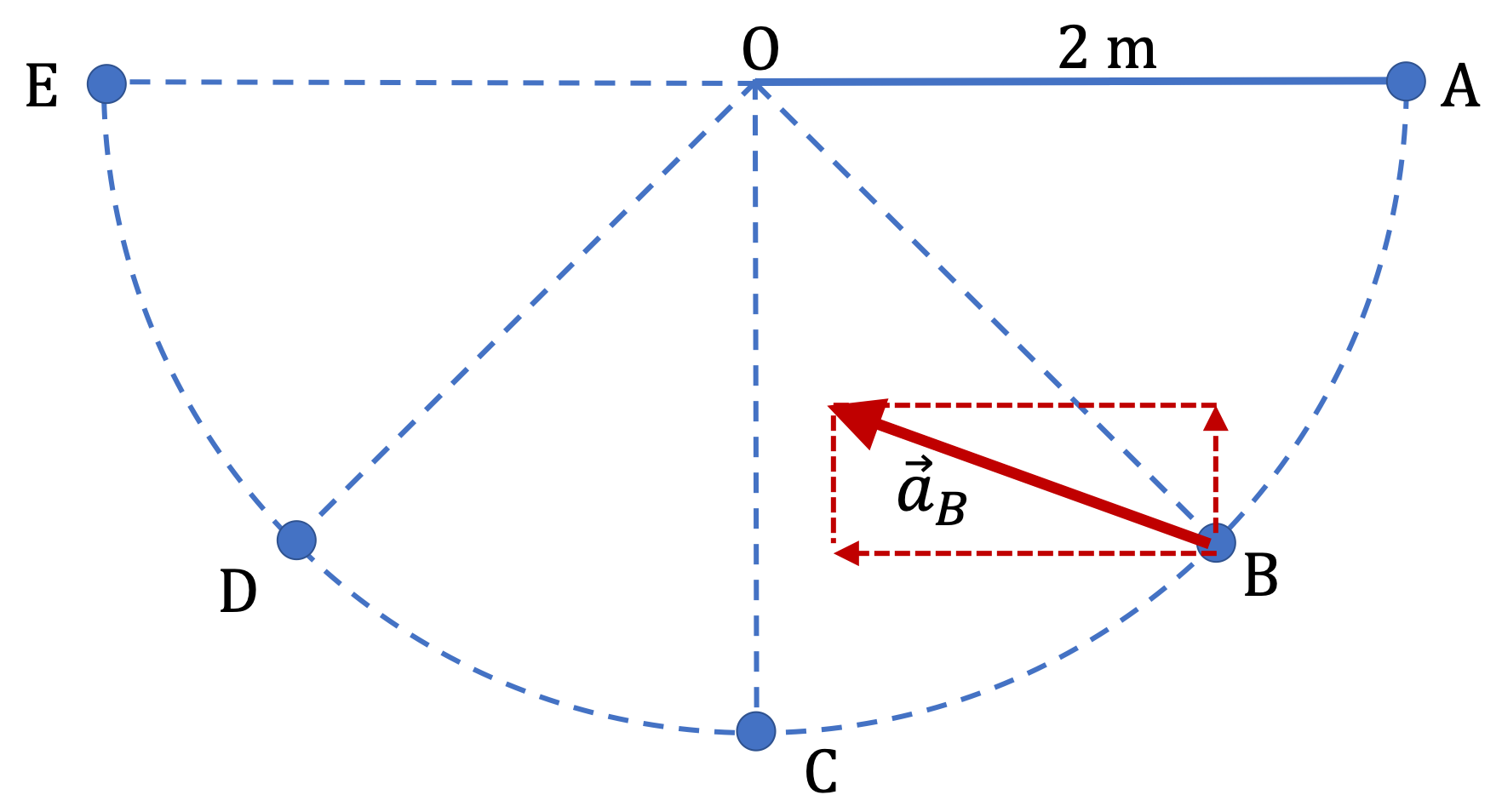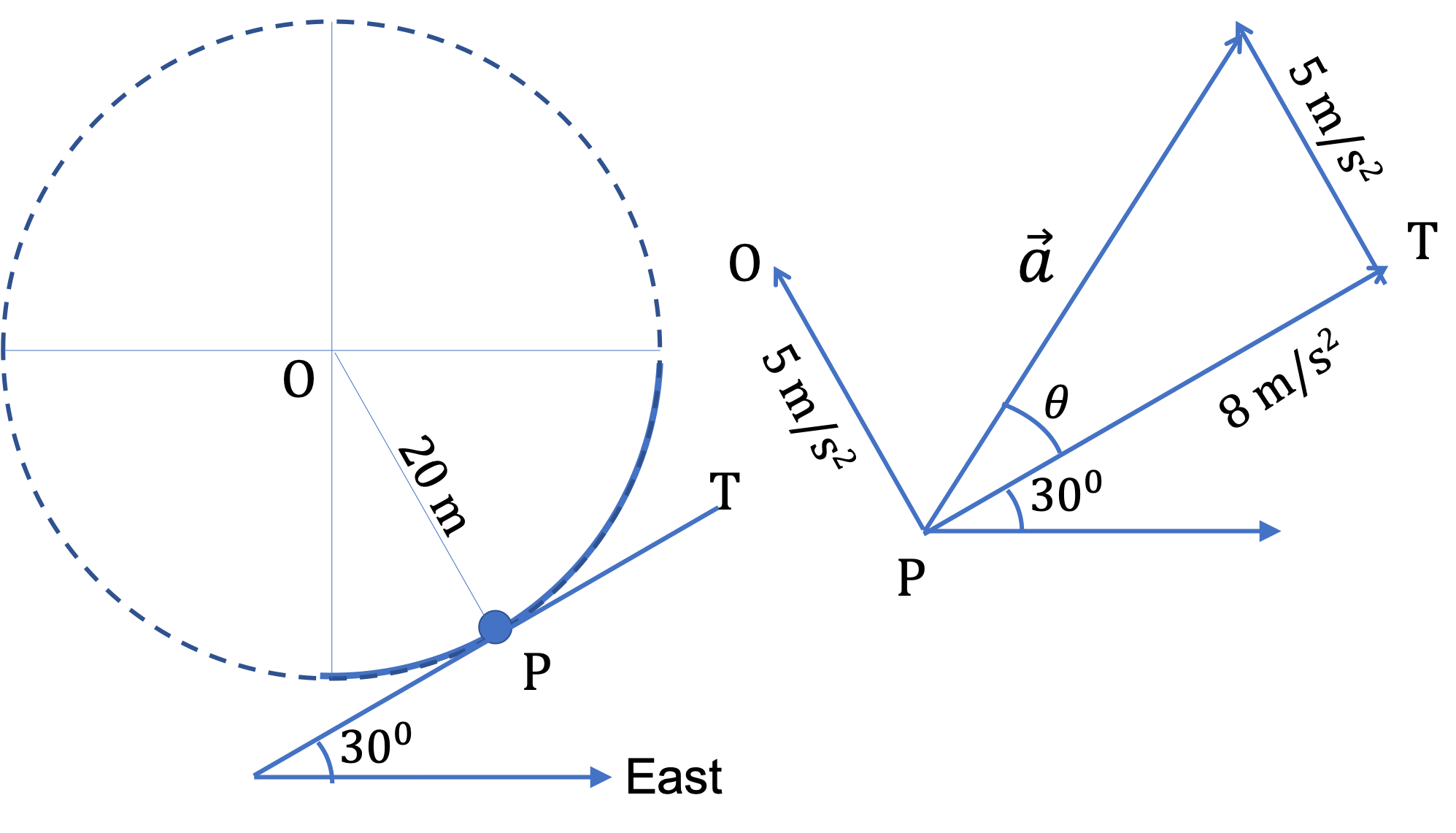## Section5.4General Circular Motion

### Subsection5.4.1Centripetal and Tangential Accelerations

In Section 5.3, we studied acceleration of a uniform circular motion. In that type of motion, speed of the particle does not change and the acceleration is pinted towards the center of the circle, which is perpendicular to the direction of velocity. This acceleration is called centripetal acceleration. Let us denote this as a vector $\vec a_c\text{.}$

When speed changes, there will be additional acceleration in the direction of velocity (in case of speeding up) or in the opposite direction of the velocity (in case of slowing down). Since velocity direction is tangential to circle, this type of acceleration is also tangential and hence its name, tangential acceleration as shown in Figure 5.4.1. We denote this as $\vec a_\theta\text{.}$ I am using $\theta$ to remind that the direction is either in the direction of the unit vector $(\hat u_\theta = -\hat i \sin\theta + \hat j \cos\theta)\text{,}$ where $\hat i$ and $\hat j$ are unit vectors towards positive $x$ and $y$ axes.Figure 5.4.1. Centripetal and tangential acceleration directions. While the centripetal acceleration is always pointed towards the center, the tangential acceleration will point in the forward direction, i.e., the same direction as the velocity, if the body is speeding up as in (a), and will point back, i.e., opposite to the velocity if it is slowing down, as in (b).

The net acceleration, i.e., acceleration, $\vec a\text{,}$ of the object in a circular motion will be a vector sum of the two types of acceleration as shown in Figure 5.4.1.

\begin{equation} \vec a = \vec a_c + \vec a_\theta.\tag{5.4.1} \end{equation}

Let us denote the magnitude of the tangential acceleration by $a_\theta$ and that of the centripetal acceleration by $a_c\text{.}$ We have already seen the formula for the centripetal acceleration,

\begin{equation} a_c = \dfrac{v^2}{R},\tag{5.4.2} \end{equation}

and the formula for the magnitude of the tangential acceleration is rate at which speed is changing

\begin{equation} a_\theta = \frac{\Delta v}{\Delta t} \longrightarrow \frac{d v}{d t}.\tag{5.4.3} \end{equation}

The magnitude of the net acceleration $\vec a$ of the body in circular motion is obtained from combining these two perpendicularly oriented components,

\begin{equation} a = \sqrt{a_c^2 + a_\theta^2} = \sqrt{ \left( v^2/R\right)^2 + \left(dv/dt \right)^2 }.\tag{5.4.4} \end{equation}

We can find the direction of net acceleration either graphically or anlaytically from the directions of the centripetal and tangential accelerations are illustrated in Fig. Figure 5.4.1.

A pendulum swings back and forth. In one of those swings, the pendulum bob goes from A to E shown in the Figure. The speed and magnitude of tangential acceleration at the points marked on the figure are

\begin{align*} \amp v_A = 0, \ \ \ |a_\theta|_A = 9.81\,\text{m/s}^2\\ \amp v_B = 5.3\,\text{ m/s}, \ \ \ |a_\theta|_B = 7\,\text{m/s}^2\\ \amp v_C = 6.3\,\text{ m/s}, \ \ \ |a_\theta|_C = 0\\ \amp v_D = 5.3\,\text{ m/s}, \ \ \ |a_\theta|_D= 7\,\text{m/s}^2\\ \amp v_E = 0, \ \ \ |a_\theta|_E = 9.81\,\text{m/s}^2 \end{align*}

Find magnitude and direction of the net acceleration at these instants. Note that direction of the swing is important here.

Hint

At each instant add the centripetal and tangential accelerations vectorially.

(A) $\vec a_A = -9.81\, \hat j,\text{,}$ (B) $-\dfrac{21}{\sqrt{2}} \, \hat i + \dfrac{7}{\sqrt{2}} \, \hat j. ,$ (C) $19.8\text{ m/s}^2\, \hat j,$ (D) $\dfrac{21}{\sqrt{2}} \, \hat i + \dfrac{7}{\sqrt{2}} \, \hat j,$ (E) $-9.81\text{ m/s}^2\, \hat j \text{.}$

Solution

We need to add the centripetal and tangential accelerations vectorially. Let's work out thee magnitude and directions of these at eqch instant first. For direction's sake, let $x$ axis be pointed to the right and $y$ pointed Up, and use the unit vectors $\hat i$ and $\hat j\text{.}$ You could also do these calculations by keeping track of $x$ and $y$ components.

A.

The centripetal and tangential acceleration vectors are:

\begin{align*} \amp a_c = 0\ \ (\text{since } v = 0.)\\ \amp a_\theta = -9.81\, \hat j. \end{align*}

Adding then, we get the net acceleration at A to be

\begin{equation*} \vec a_A = -9.81\, \hat j. \end{equation*}

B.

The magnitude of the centripetal acceleration is

\begin{equation*} a_c = \dfrac{v^2}{R} = \dfrac{5.3^2}{2} = 14.0\text{ m/s}^2. \end{equation*}

The direction is in the second quadrant in the following unit vector direction.

\begin{equation*} \hat u_c = -\cos\, 45^{\circ}\, \hat i + \sin\, 45^{\circ}\, \hat j = \dfrac{-\hat i + \hat j}{\sqrt{2}} \end{equation*}

Similarly, the tangential direction in pointed in the third quadrant.

\begin{equation*} \hat u_\theta = -\cos\, 45^{\circ}\, \hat i - \sin\, 45^{\circ}\, \hat j = \dfrac{-\hat i - \hat j}{\sqrt{2}} \end{equation*}

Therefore, the centripetal and tangential accelerations vectors in the component for are:

\begin{align*} \amp a_c = \dfrac{14.0}{\sqrt{2}} \left( -\hat i + \hat j\right)\\ \amp a_\theta = \dfrac{7}{\sqrt{2}}\left( -\hat i - \hat j\right). \end{align*}

Adding then, we get the net acceleration at A to be

\begin{equation*} \vec a_B= -\dfrac{21}{\sqrt{2}} \, \hat i + \dfrac{7}{\sqrt{2}} \, \hat j. \end{equation*}

That is the acceleration has the magnitude

\begin{equation*} \sqrt{ \dfrac{21^2}{2} + \dfrac{7^2}{2} } \text{ m/s}^2 = 15.65\,\text{m/s}^2. \end{equation*}

The direction of acceleration is shown in the figure.C.

The tangential acceleration here is zero, so the net acceleration is just the centripetal acceleration, which is directed vertically up. Therefore,

\begin{equation*} \vec a_C = \dfrac{v^2}{R}\, \hat j = 19.8\, \hat j. \end{equation*}

That is, the magnitude is $19.8\text{ m/s}^2\text{.}$

D.

Now, compared to ppoint B, the pendulum bob is slowing. Therefore, tangential acceleration is pointed in the fourth quadrant and the centripetal acceleration, being radial, is pointed in the first quadrant. Draw figures to convince youerself. This will mean that we will have net acceleration towards positive $x$ direction and same magnitude as in B.

\begin{equation*} \vec a_D = \dfrac{21}{\sqrt{2}} \, \hat i + \dfrac{7}{\sqrt{2}} \, \hat j. \end{equation*}

E.

The situation here is same as A since centripetal acceleration is zero and tangential is pointed in the vertically down direction.

\begin{equation*} \vec a_E = -9.81\text{ m/s}^2\, \hat j. \end{equation*}

A car is taking a left turn as shown in Figure 5.4.5. While in the turn, car moves on a circular arc of radius $20\text{ m}\text{,}$ but its speed is increasing at a steady pace of $8\text{ m/s}^2$ such that at the location marked P, speed is $10\text{ m/s}\text{.}$

(a) Find magnitudes of centripetal acceleration, tangential acceleration, and full acceleration of the car.

(b) If tangent to the arc at point P is pointed in the direction $30^{\circ}$ North of East, what is the direction of acceleration at this instant?

Hint

(a) Use formula. (b) Use the fact that tangential and centripetal directions are perpendicular to each other.

(a) $5\text{ m/s}^2,\ 8\text{ m/s}^2,\ 9.43\text{ m/s}^2\text{,}$ (b) $62^{\circ}$ North of East.

Solution

(a) The tangential acceleration is already given in the problem statement.

\begin{align*} \amp a_c = \dfrac{v^2}{R} = \dfrac{10^2}{20} = 5\text{ m/s}^2,\\ \amp a_\theta = 8\text{ m/s}^2,\\ \amp a = \sqrt{a_c^2 + a_\theta^2} = \sqrt{5^2 + 8^2} = 9.43\text{ m/s}^2. \end{align*}

(b) A figure will help.Once we find angle $\theta$ in the figure, we can add $30^{\circ}$ to it to get the direction from the East.

\begin{equation*} \theta = \tan^{-1}\left(\dfrac{5}{8} \right) = 32^{\circ}. \end{equation*}

Therefore, the direction of acceleration at this instant is $32^{\circ} + 30^{\circ} = 62^{\circ}$ North of East.

### Subsection5.4.2(Calculus) Velocity and Speed for a Circular Motion

Velocity is derivative of position. Since $\theta$ is the only thing changing in a circular motion, the rate of change of $\theta$ will figure prominantly in the velocity. We call the rate of change of $\theta$ the angular velocity, and denote it by $\omega \text{,}$ the small “omega”.

\begin{equation} \text{Angular velocity: }\ \ \ \ \omega = \dfrac{d\theta}{dt}.\tag{5.4.5} \end{equation}

Let's perform the derivatives component by component. Thus, $x$ and $y$ components of the velocity will be

\begin{align*} v_x \amp = \dfrac{dx}{dt} = \dfrac{d}{dt} (R\, \cos\, \theta(t)) = -R\, \sin\, \theta(t)\, \dfrac{d\theta}{dt} = - y\, \omega \\ v_y \amp = \dfrac{dy}{dt} = \dfrac{d}{dt} (R\, \sin\, \theta(t)) = R\, \cos\, \theta(t)\, \dfrac{d\theta}{dt} = x\, \omega \end{align*}

The magnitude of the velocity, i.e., speed is

\begin{equation*} v = \sqrt{v_x^2 + v_y^2} = \sqrt{ \left( y^2 + x^2 \right) \omega^2 } =\sqrt{R^2\omega^2} = R |\omega|, \end{equation*}

and the tangent of the angle of velocity with respect to the $x$ axis is

\begin{equation*} \tan\theta_{v} = \left(\dfrac{v_y}{v_x} \right) = \left(\dfrac{x\, \omega}{- y\, \omega} \right) = \left( \dfrac{\cos\,\theta}{- \sin\,\theta} \right). \end{equation*}

The right side can be rewritten as

\begin{equation*} \left( \dfrac{\cos\,\theta}{- \sin\,\theta} \right) = \tan\left( \theta \pm \dfrac{\pi}{2}\right), \end{equation*}

where plus is for the counterclockwise motion and negative for the clockwise motion. We will pick the counterclockwise motion. Therefore, the angle of the velocity vector with respect to the $x$ axis is

\begin{equation} \theta_v = \theta + \dfrac{\pi}{2},\tag{5.4.6} \end{equation}

where $\theta$ is the angle on the circle modulu $2\pi\text{.}$

Let's summarize the results.

\begin{align*} \amp\text{Components: } v_x = - y\, \omega,\ \ v_y = - x\, \omega,\\ \amp\text{Magnitude, i.e., Speed: } v = R |\omega|,\\ \amp\text{Direction for counterclockwise motion: } \theta_v = \theta + \dfrac{\pi}{2}, \end{align*}

where $\theta$ is the angle on the circle modulu $2\pi$ and $\theta_v$ is angle with respect to the $x$ axis. After we find the value of $\theta_v\text{,}$ we need to interpret the value for the direction based on the quadrant to which point $(v_x, v_y)$ belongs.

### Subsection5.4.3(Calculus) Acceleration of a Circular Motion

Acceleration is derivative of velocity. Here, we will need to quantify the rate at which the angular velocity $\omega$ changes. This is called angular acceleration, which we will denote by the symbol $\alpha\text{.}$

\begin{equation} \alpha = \dfrac{d\omega}{dt} = \dfrac{d^2\theta}{dt^2}.\tag{5.4.7} \end{equation}

We will see below that $R\alpha$ is the tangential acceleration.

\begin{equation*} R\alpha = R\dfrac{d\omega}{dt} = \dfrac{d(R\omega)}{dt} = \dfrac{dv}{dt}. \end{equation*}

Acceleration, i.e., vector $\vec a \text{,}$ is the rate of change of the velocity vector $\vec v \text{.}$ Its much easier to work this out using Cartesian components.

\begin{align*} a_x \amp = \dfrac{dv_x}{dt} = - \dfrac{d}{dt}(y\, \omega) = -v_y\,\omega - y\, \alpha\\ a_y \amp = \dfrac{dv_y}{dt} = \dfrac{d}{dt} (x\, \omega) = v_x\, \omega + x\, \alpha \end{align*}

The magnitude of the acceleration vector is

\begin{equation} a = \sqrt{a_x^2 + a_y^2} = \sqrt{ \left( \dfrac{v^2}{R}\right)^2 + (R\alpha)^2 },\label{eq-circ-motion-acceleration-magnitude}\tag{5.4.8} \end{equation}

where simplification occurs in the detailed calculation by noting that

\begin{equation*} x v_x + y v_y = 0, \end{equation*}

which is obtained by taking derivative of $x^2 + y^2 = R^2\text{,}$ and replacing $\omega$ by $v /R\text{.}$ You should work out the full derivation of the formula for $a$ in Eq. (5.4.8).

The full acceleration in Eq. (5.4.8) has two contributing parts, which come from perpendicular directions. The first part, $\dfrac{v^2}{R}$ is the centripetal acceleration, which is due to changing direction of velocity, and the second part $R\alpha\text{,}$ which can also be written as $dv/dt\text{,}$ is called the tangential acceleration. We will denote centripetal acceleration by $a_c$ and the tangential by $a_\theta\text{.}$ (The use of subscript $\theta$ will be come clear if/when you do the polar coordinates.)

\begin{align} \amp \text{Centripetal Acceleration: } a_c = \dfrac{v^2}{R},\tag{5.4.9}\\ \amp \text{Tangential Acceleration: } a_\theta = |R\alpha|= \left| \dfrac{dv}{dt} \right|. \tag{5.4.10} \end{align}

The directions of centripetal and tangential accelerations are shown in Fig. Figure 5.4.1.